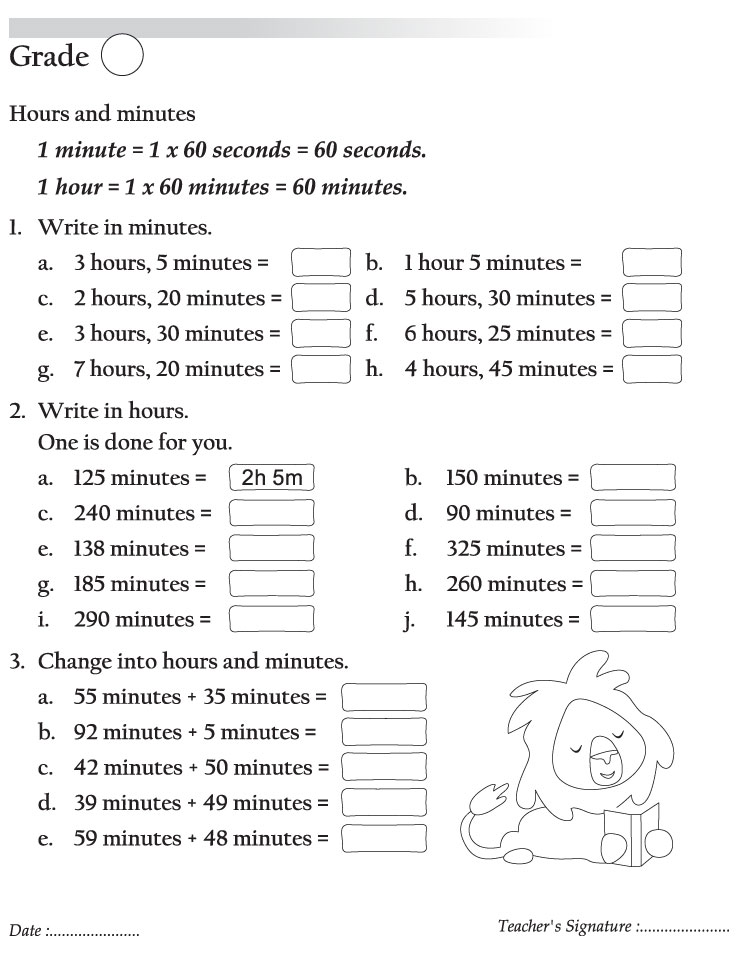# Home

## Worksheet Category

### Hours and minutes

Page of 56Page of 56Numbers in Ascending OrderNumbers in Descending OrderOrder of numbersMissing NumbersExpanded and contracted forms of numbersPlace ValueIncreasing, decreasing and combination of numbersEven and odd numbersSmallest and highestHindu-Arabic and Roman numeralsHindu-Arabic and Roman numeralsAdditionAddition related questionsAddition circleMore additionSubtractionMore subtractionSubtraction related questionsMultiplicationMultiplication related questionsMultiplication tablesDivisionDivision tableDivision related questionsFractionsFractionsFractions of divisionMore fractionsFractions of shaded and unshaded partsGeomatrical shapesMore geomatrical shapesPerimeter of geometrical shapesTime: A.M. and P.M.Write sown time as shown in the clockDraw hands to indicate the time as written below the each clockHours and minutesTime related questionsTime related quizIndian currencyConversion of moneyMoney: Addition and subtractionMoney related questionsMeasurement of length�Unit of lengthConversion of lengthLength: Addition and subtractionLength related questionsMeasurement of capacityCapacity: Addition and subtractionCapacity related questionsMeasurement of mass unitsConversion of weightsWeight related questionsCalendarMisc. questions�capacityLong number names